The next operation that follows magnitude is normalization. Normalization consists of dividing every entry in a vector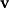by its magnitude to create a vector of length 1 known as the unit vector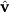(pronounced "v-hat").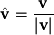For example, the vector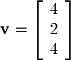has magnitude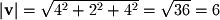. It's unit vector is given by the following: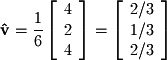Figure 2-6 shows thatis made up of 6 unit vectors. One can also easily see that normalization changes the magnitude to 1 but leaves the direction unchanged.
Object could not be loaded.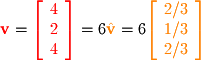Figure 2-6.
Vector and its Unit Norm
An important application of normalization is to rescale a vector to a particular magnitude without changing its direction. If we take the same vectorabove with magnitude 6 and want to give it a magnitude of 9 we simply multiply 9 by the unit vector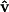: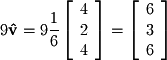Excercise 2-4.
1.   Find the norm of the vector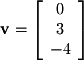2.   Find the vector in the same direction as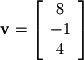with magnitude 3.